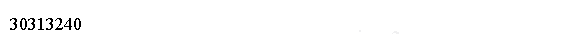# OCA Java SE 8 Operators/Statements - Java Operators

A Java operator can be applied to a set of variables, values, or literals (operands) and that returns a result.

Three kinds of operators are available in Java:

• unary,
• binary, and
• ternary.

These types of operators can be applied to one, two, or three operands, respectively.

Java operators are not necessarily evaluated from left-to-right order.

For example, the following Java expression is actually evaluated from right-to-left given the specific operators involved.

```public class Main{
public static void main(String[] argv){
int y = 4;
double x = 5 + 2 * --y;
System.out.println(x);
System.out.println(y);
}
}
```

In this example, you would first decrement y to 3, and then multiply the resulting value by 2, and finally add 5.

The value would then be automatically upcast from int to double and assigned to x.

## Order

Java operators follow order of operation listed in the following table, by decreasing order of operator precedence.

If two operators have the same level of precedence, then Java guarantees left-to-right evaluation.

You need to know only those operators in bold for the OCA exam.

OperatorSymbols and examples
Post-unary operatorsexpression++, expression--
Pre-unary operators++expression, --expression
OperatorSymbols and examples
Other unary operators+, -, !
Multiplication/Division/Modulus*, /, %
Shift operators<<, >>, >>>
Relational operators<, >, <=, >=, instanceof
Equal to/not equal to==, !=
Logical operators&, ^, |
Short-circuit logical operators&&, ||
Ternary operatorsboolean expression ? expression1 : expression2
Assignment operators=, +=, -=, *=, /=, %=, &=, ^=, !=, <<=, >>=, >>>=

## Binary Arithmetic Operators

The binary operators perform mathematical operations on variables, create logical expressions, as well as perform basic variable assignments.

Binary operators are commonly combined in complex expressions with more than two variables.

## Arithmetic Operators

Arithmetic operators include addition (+), subtraction (-), multiplication (*), division (/), and modulus (%).

They also include the unary operators, ++ and --.

The multiplicative operators (*, /, %) have a higher order of precedence than the additive operators (+, -).

```public class Main{
public static void main(String[] argv){
int x = 2 * 5 + 3 * 4 - 8;
System.out.println(x);
}
}
```

We can use the parentheses to change the order of operation explicitly by wrapping parentheses around the sections you want evaluated first.

All of the arithmetic operators may be applied to any Java primitives, except boolean and String.

Only the addition operators + and += may be applied to String values, which results in String concatenation.

## modulus operator

The modulus %, or remainder operator, gets the remainder when two numbers are divided.

For example, 9 divided by 3 divides evenly and has no remainder; therefore 9 % 3 is 0.

11 divided by 3 does not divide evenly; therefore, the remainder, or 11 % 3, is 2.

```public class Main{
public static void main(String[] argv){
System.out.print(9 / 3);  // Outputs 3
System.out.print(9 % 3);  // Outputs 0
System.out.print(10 / 3);  // Outputs 3
System.out.print(10 % 3);  // Outputs 1
System.out.print(11 / 3);  // Outputs 3
System.out.print(11 % 3);  // Outputs 2
System.out.print(12 / 3);  // Outputs 4
System.out.print(12 % 3);  // Outputs 0
}/*  w w w .j  a v  a2s.  co  m*/
}
```

The modulus operation is not limited to positive integer values in Java and may also be applied to negative integers and floating-point integers.

For a given divisor y and negative dividend, the resulting modulus value is between and (-y + 1) and 0.

The code above generates the following result.